# RD Sharma Solutions For Class 7 Maths Chapter 11 Percentage Exercise 11.6

Students can view and download the PDF of RD Sharma Solutions for Class 7 Chapter 11 Percentage Exercise 11.6 available here. Our specialist teachers formulate these exercises to help you with your exam preparation to achieve good marks in Maths. The solutions are stepwise and detailed to make learning easy for students. This exercise has fifteen verbally based questions based on the topic of percentage to solve real-life problems. It also deals with an increase or decrease in percent. To know more about these topics it is advised to solve RD Sharma Solutions for Class 7.

## Download the PDF of RD Sharma Solutions For Class 7 Maths Chapter 11 – Percentage Exercise 11.6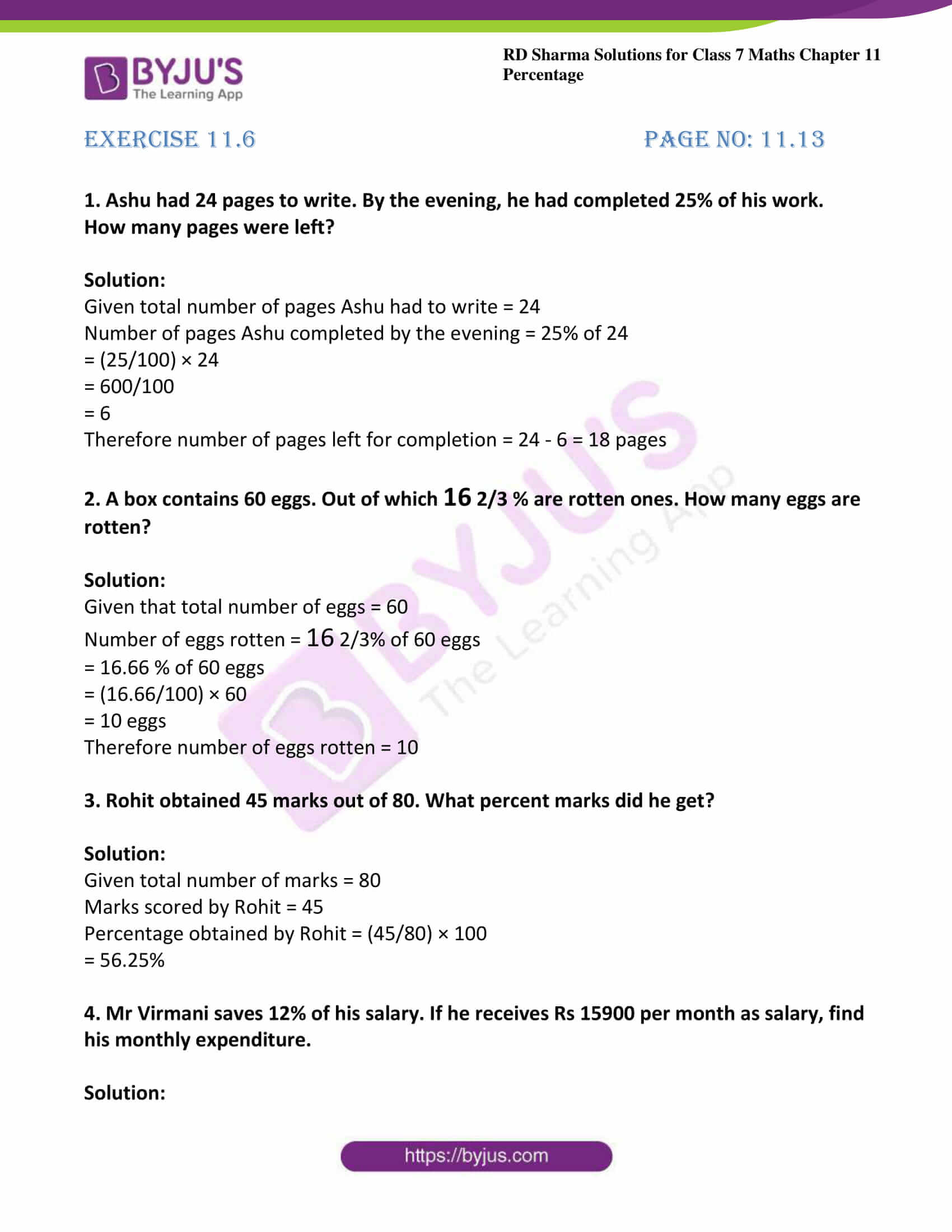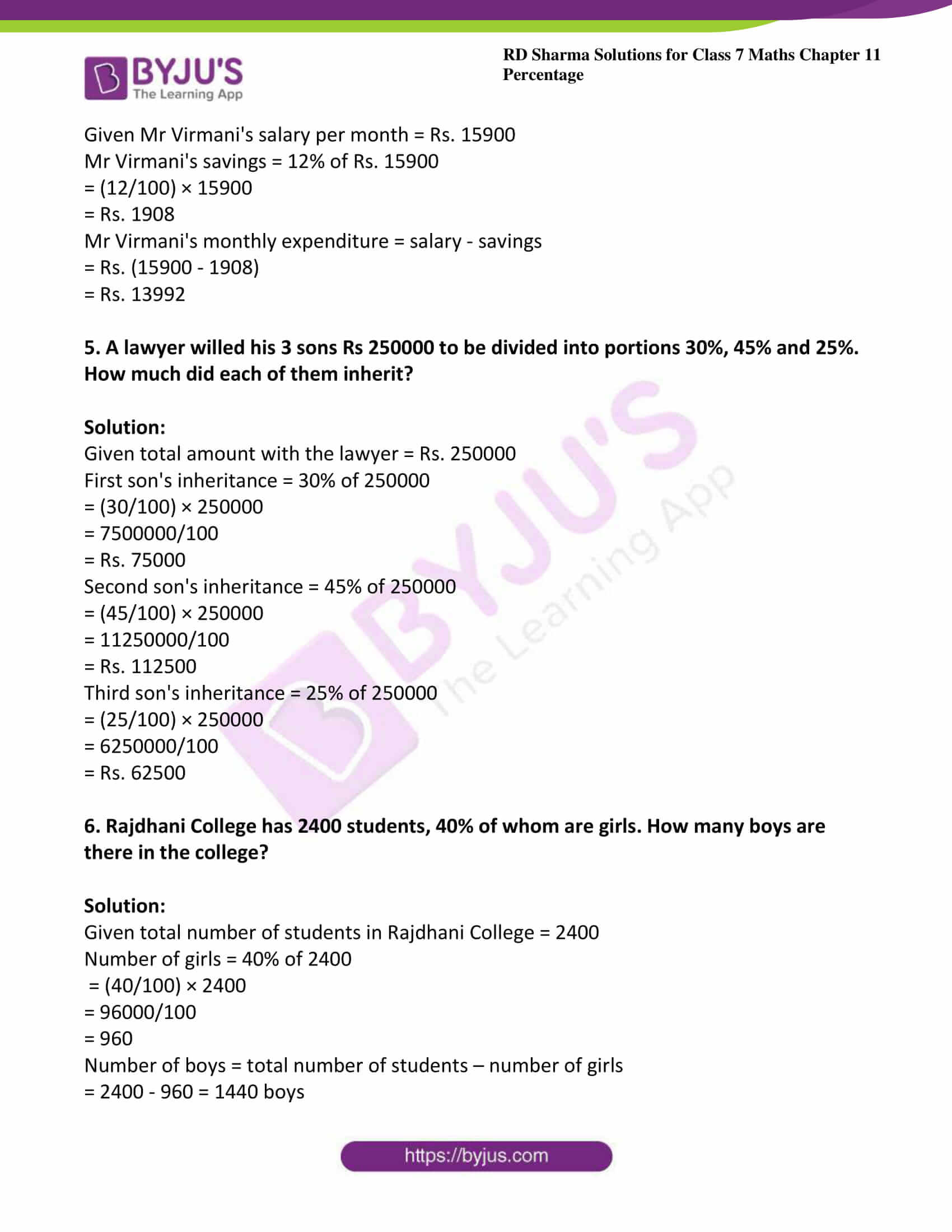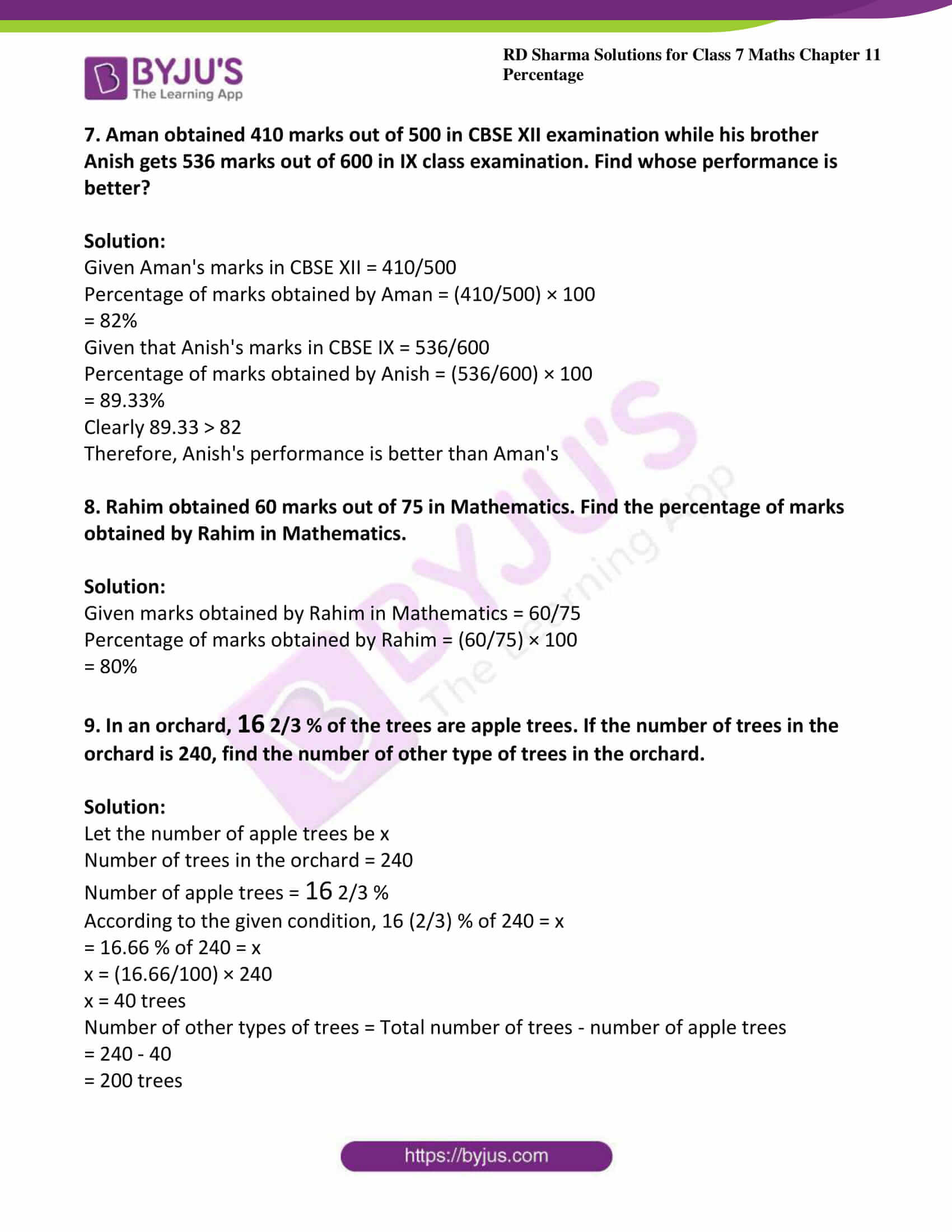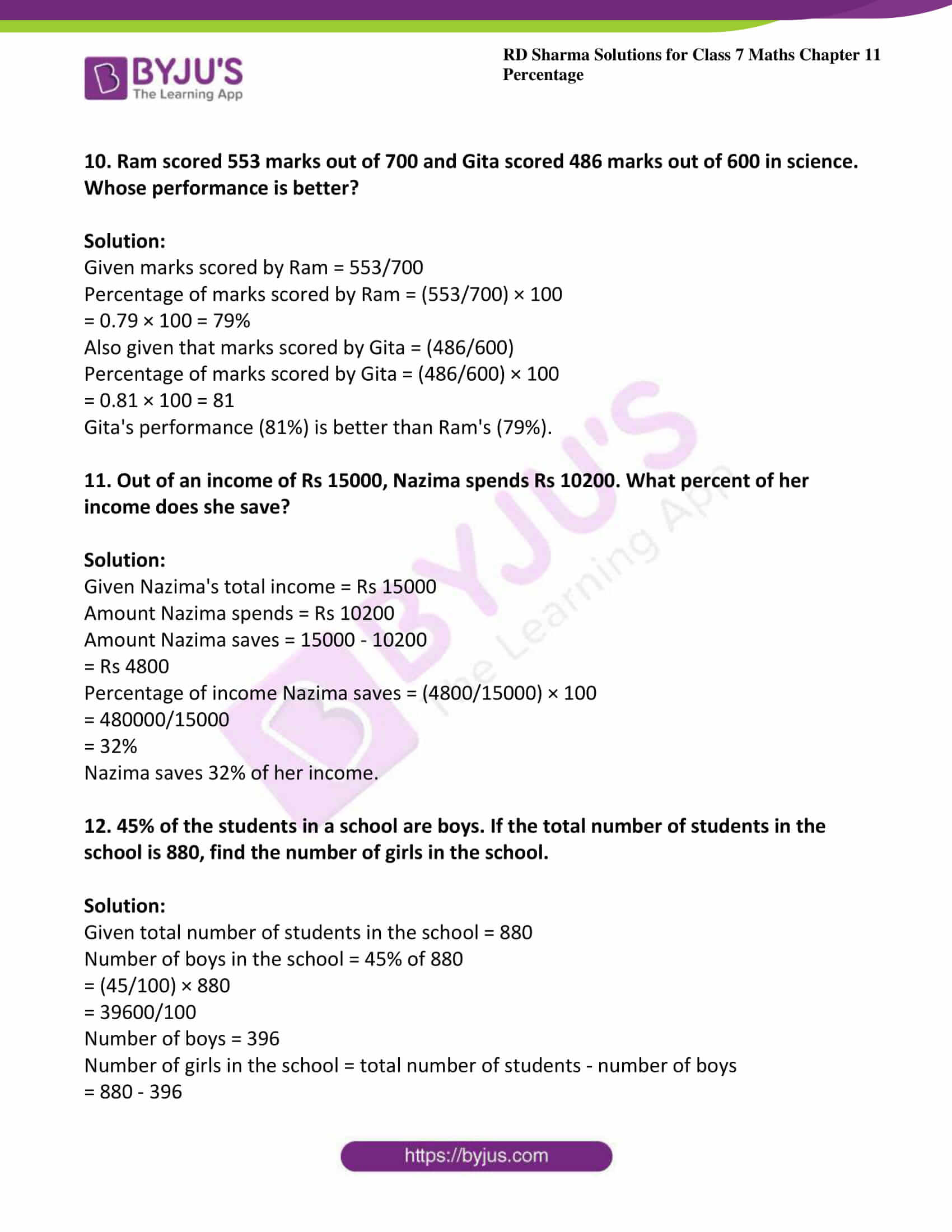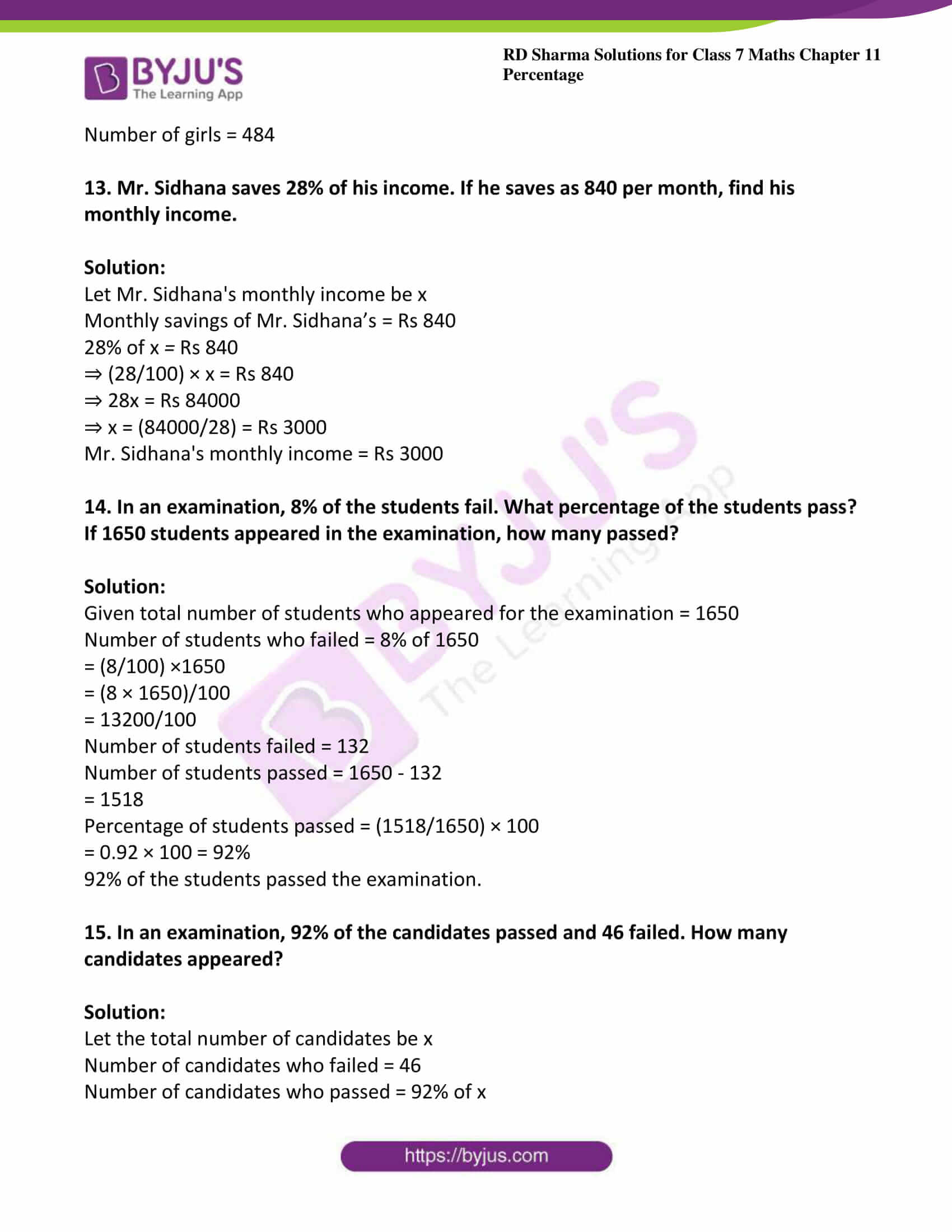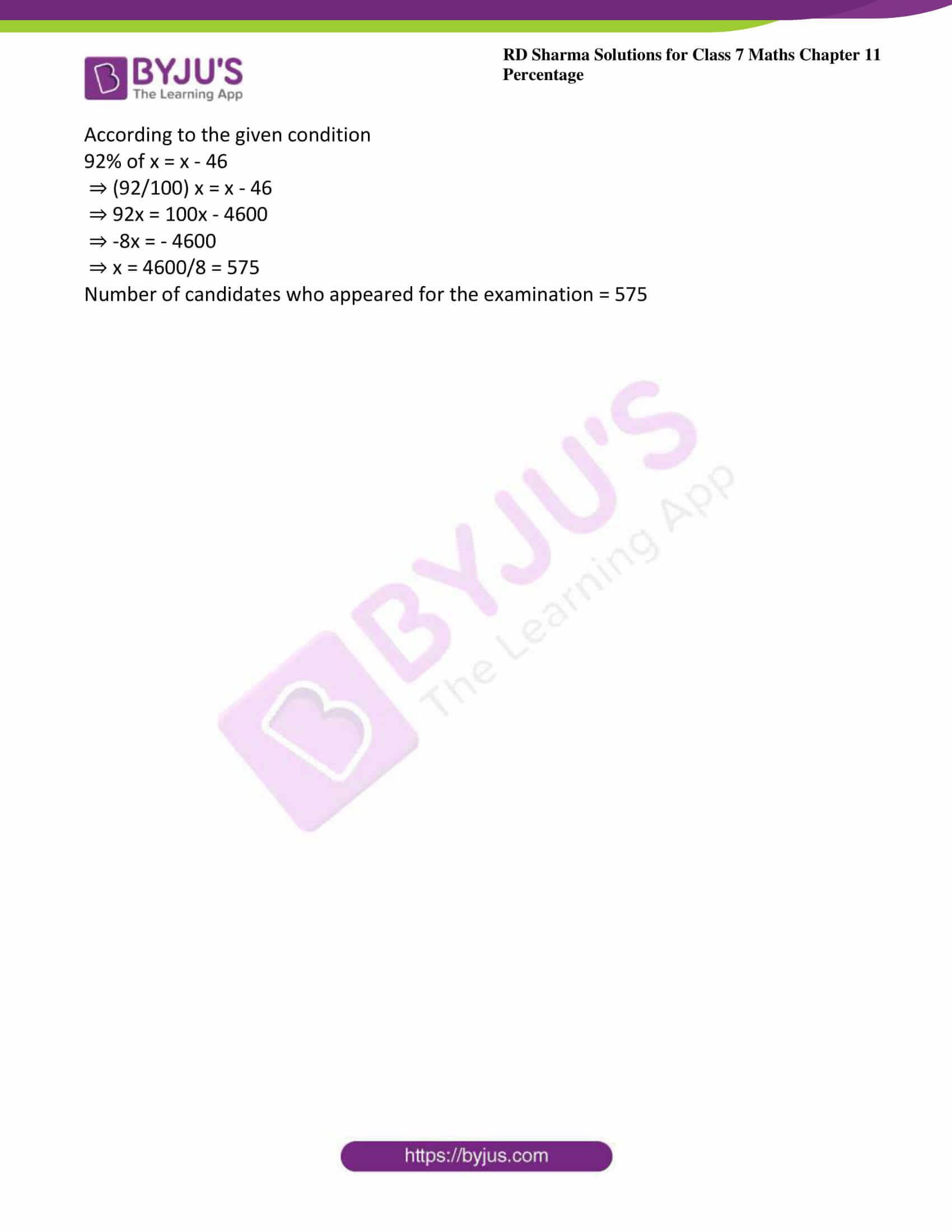### Access answers to Maths RD Sharma Solutions For Class 7 Chapter 11 – Percentage Exercise 11.6

Exercise 11.6 Page No: 11.13

1. Ashu had 24 pages to write. By the evening, he had completed 25% of his work. How many pages were left?

Solution:

Given total number of pages Ashu had to write = 24

Number of pages Ashu completed by the evening = 25% of 24

= (25/100) × 24

= 600/100

= 6

Therefore number of pages left for completion = 24 – 6 = 18 pages

2. A box contains 60 eggs. Out of which 16 2/3 % are rotten ones. How many eggs are rotten?

Solution:

Given that total number of eggs = 60

Number of eggs rotten = 16 2/3% of 60 eggs

= 16.66 % of 60 eggs

= (16.66/100) × 60

= 10 eggs

Therefore number of eggs rotten = 10

3. Rohit obtained 45 marks out of 80. What percent marks did he get?

Solution:

Given total number of marks = 80

Marks scored by Rohit = 45

Percentage obtained by Rohit = (45/80) × 100

= 56.25%

4. Mr Virmani saves 12% of his salary. If he receives Rs 15900 per month as salary, find his monthly expenditure.

Solution:

Given Mr Virmani’s salary per month = Rs. 15900

Mr Virmani’s savings = 12% of Rs. 15900

= (12/100) × 15900

= Rs. 1908

Mr Virmani’s monthly expenditure = salary – savings

= Rs. (15900 – 1908)

= Rs. 13992

5. A lawyer willed his 3 sons Rs 250000 to be divided into portions 30%, 45% and 25%. How much did each of them inherit?

Solution:

Given total amount with the lawyer = Rs. 250000

First son’s inheritance = 30% of 250000

= (30/100) × 250000

= 7500000/100

= Rs. 75000

Second son’s inheritance = 45% of 250000

= (45/100)​ × 250000

= 11250000/100

= Rs. 112500

Third son’s inheritance = 25% of 250000

= (25/100)​ × 250000

= 6250000/100

= Rs. 62500

6. Rajdhani College has 2400 students, 40% of whom are girls. How many boys are there in the college?

Solution:

Given total number of students in Rajdhani College = 2400

Number of girls = 40% of 2400

= (40/100) × 2400

= 96000/100

= 960

Number of boys = total number of students – number of girls

= 2400 – 960 = 1440 boys

7. Aman obtained 410 marks out of 500 in CBSE XII examination while his brother Anish gets 536 marks out of 600 in IX class examination. Find whose performance is better?

Solution:

Given Aman’s marks in CBSE XII = 410/500

Percentage of marks obtained by Aman = (410/500) × 100

= 82%

Given that Anish’s marks in CBSE IX = 536/600

Percentage of marks obtained by Anish = (536/600) × 100

= 89.33%

Clearly 89.33 > 82

Therefore, Anish’s performance is better than Aman’s

8. Rahim obtained 60 marks out of 75 in Mathematics. Find the percentage of marks obtained by Rahim in Mathematics.

Solution:

Given marks obtained by Rahim in Mathematics = 60/75

Percentage of marks obtained by Rahim = (60/75) × 100

= 80%

9. In an orchard, 16 2/3 % of the trees are apple trees. If the number of trees in the orchard is 240, find the number of other type of trees in the orchard.

Solution:

Let the number of apple trees be x

Number of trees in the orchard = 240

Number of apple trees = 16 2/3 %

According to the given condition, 16 (2/3) % of 240 = x

= 16.66 % of 240 = x

x = (16.66/100) × 240

x = 40 trees

Number of other types of trees = Total number of trees – number of apple trees

= 240 – 40

= 200 trees

10. Ram scored 553 marks out of 700 and Gita scored 486 marks out of 600 in science. Whose performance is better?

Solution:

Given marks scored by Ram = 553/700

Percentage of marks scored by Ram = (553/700) × 100

= 0.79 × 100 = 79%

Also given that marks scored by Gita = (486/600)

Percentage of marks scored by Gita = (486/600) × 100

= 0.81 × 100 = 81

Gita’s performance (81%) is better than Ram’s (79%).

11. Out of an income of Rs 15000, Nazima spends Rs 10200. What percent of her income does she save?

Solution:

Given Nazima’s total income = Rs 15000

Amount Nazima spends = Rs 10200

Amount Nazima saves = 15000 – 10200

= Rs 4800

Percentage of income Nazima saves = (4800/15000) × 100

= 480000/15000

= 32%

Nazima saves 32% of her income.

12. 45% of the students in a school are boys. If the total number of students in the school is 880, find the number of girls in the school.

Solution:

Given total number of students in the school = 880

Number of boys in the school = 45% of 880

= (45/100) × 880

= 39600/100

Number of boys = 396

Number of girls in the school = total number of students – number of boys

= 880 – 396

Number of girls = 484

13. Mr. Sidhana saves 28% of his income. If he saves as 840 per month, find his monthly income.

Solution:

Let Mr. Sidhana’s monthly income be x

Monthly savings of Mr. Sidhana’s = Rs 840

28% of xRs 840

⇒ (28/100) × x = Rs 840

⇒ 28x = Rs 84000

⇒ x = (84000/28) = Rs 3000

Mr. Sidhana’s monthly income = Rs 3000

14. In an examination, 8% of the students fail. What percentage of the students pass? If 1650 students appeared in the examination, how many passed?

Solution:

Given total number of students who appeared for the examination = 1650

Number of students who failed = 8% of 1650

= (8/100) ×1650

= (8 × 1650)/100

= 13200/100

Number of students failed = 132

Number of students passed = 1650 – 132

= 1518

Percentage of students passed = (1518/1650) × 100

= 0.92 × 100 = 92%

92% of the students passed the examination.

15. In an examination, 92% of the candidates passed and 46 failed. How many candidates appeared?

Solution:

Let the total number of candidates be x

Number of candidates who failed = 46

Number of candidates who passed = 92% of x

According to the given condition

92% of x = x – 46

⇒ (92/100) x = x – 46

⇒ 92x = 100x – 4600

⇒ -8x = – 4600

⇒ x = 4600/8 = 575

Number of candidates who appeared for the examination = 575